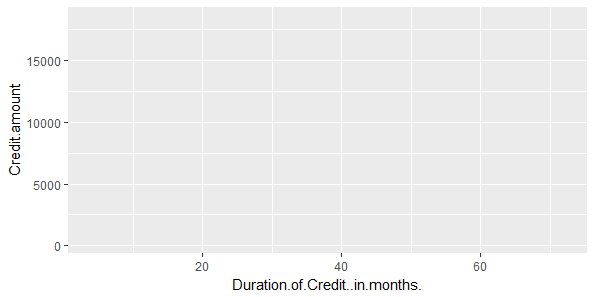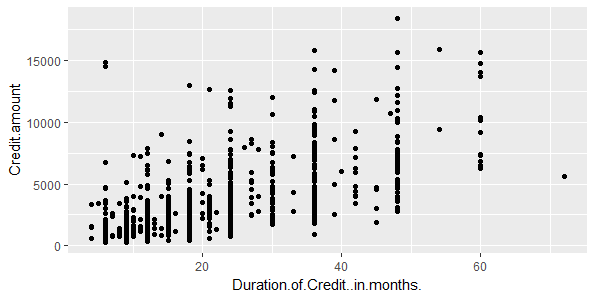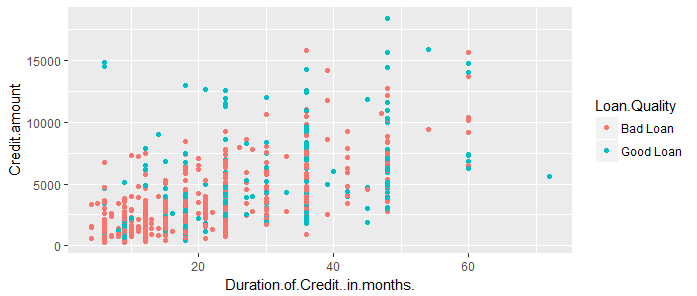# Create ggplot Graph with German Credit Data in R

In ggplot2 package, we use the ggplot() function to create a fully customized data visualization. We still have the German credit data loaded in the dataframe df.

We will start by plotting a simple scatter graph that plots the duration of credit on x-axis and the amount of credit on y-axis. We can do so using the following command.

> ggplot(df,aes(x=Duration.of.Credit..in.months.,y=Credit.amount))

or

g <- ggplot(df,aes(x=Duration.of.Credit..in.months.,y=Credit.amount))


The process of creating a graph starts with the ggplot() function. Note that we can either directly issue the command which will print the graph or we can create an object by assigning the function to a variable. In the 2nd example above, we have created an R object called g that stores the graph object.

The above command uses only the first two levels of the grammar of graphics, i.e., data and aesthetics. If we print this, it will only print an empty graph, as shown below:We use the third level, that is, geom (geometric object) to print the actual data on the graph. Geometric objects are the actual marks we put on a plot.

• points (geom_point, for scatter plots, dot plots, etc)
• lines (geom_line, for time series, trend lines, etc)
• boxplot (geom_boxplot, for, well, boxplots!)

We use geom_points to create scatter plots, geom_bar for bar chart and so on. A plot must have at least one geom; there is no upper limit. You can add a geom to a plot using the + operator.

You can get a list of available geometric objects using the code below:

help.search("geom_", package = "ggplot2")


### Points (Scatterplot)

We now know the data that we want to plot, the aesthetics, and the geometric object that we want to create. We can use this information to complete our scatter plot.

We will add the points geom to our graph object g, as shown below:

> g <- ggplot(df,aes(x=Duration.of.Credit..in.months.,y=Credit.amount))
> g + geom_point()


The scatter plot will be created as shown below:The above scatter plot shows the relationship between the duration of credit in months and the amount loan. Each point represents a loan. In our dataset, we have 1000 loans and we also know that each loan has either defaulted or not. This is represented in the data point Loan.Quality. We can use this variable to improve this graph, for example, by coloring the points based on the loan quality.

However, there is one small problem here. The variable Loan.Quality is of type integer (1 for Bad Loan, and 2 for Good Loan). So, to use to to categorize data, we can convert it to Factor and then add levels.

#Convert Loan.Quality to Factor
df$Loan.Quality <- as.factor(df$Loan.Quality)
levels(df$Loan.Quality) <- c("Bad Loan", "Good Loan")  We can now use this variable to color the points. For this we will add the parameter color to aesthetics. > g <- ggplot(df,aes(x=Duration.of.Credit..in.months.,y=Credit.amount)) > g+geom_point(aes(color=Loan.Quality))As you can see, the points in the scatter plot are of two colors, red for bad loans, and blue for good loans. ggplot2 has automatically selected the colors and also added the legend for convenience. #### Course Downloads Get smart about tech at work. As a non-technical professional, learn how software works with simple explanations of tech concepts. Learn more... # Data Science for Finance Bundle: 43% OFF Get our Data Science for Finance Bundle for just$29 $51. Get it now for just$29
Get our Data Science for Finance Bundle for just $29$51. That's 43% OFF.
Get it for $51$29